### IMO Shortlist 2000 problem G3

Kvaliteta:
Avg: 3,5
Težina:
Avg: 6,5
Let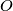$O$ be the circumcenter and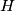$H$ the orthocenter of an acute triangle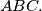$ABC.$ Show that there exist points$D, E,$ and$F$ on sides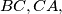$BC,CA,$ and$AB$ respectively such that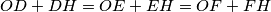and the lines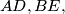$AD, BE,$ and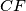$CF$ are concurrent.
Izvor: Međunarodna matematička olimpijada, shortlist 2000